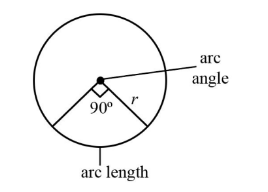The measure of an arc of a circle isand its length is. What is the diameter of the circle?

# The measure of an arc of a circle isand its length is. What is the diameter of the circle?

1. A
21cm
2. B
7cm
3. C
14cm
4. D
28cm

Fill Out the Form for Expert Academic Guidance!l

+91

Live ClassesBooksTest SeriesSelf Learning

Verify OTP Code (required)

### Solution:

The arc angle of a circle is.
The length is given as.
The length is equal to the circumference of the circle.The formula to find the circumference of the circle is equal to the pi times diameter and the ratio of arc angle and.The formula for diameter is equal to twice the radius. Since, we know that the formula for circumference of a circle is,Substituting the values of arc angle and circumference of the circle we obtain,Now, we will substitute the value of circumference of the circle in the above equation.On further solving the above value we will get the diameter of the circle which is,The diameter of the circle is approximately equal toTherefore, the diameter of the circle for the given problem is.
Hence the correct
Ans: is Option 3.

## Related content

 Area of Square Area of Isosceles Triangle Pythagoras Theorem Triangle Formula Perimeter of Triangle Formula Area Formulae Volume of Cone Formula Matrices and Determinants_mathematics Critical Points Solved Examples Type of relations_mathematics+91

Live ClassesBooksTest SeriesSelf Learning

Verify OTP Code (required)